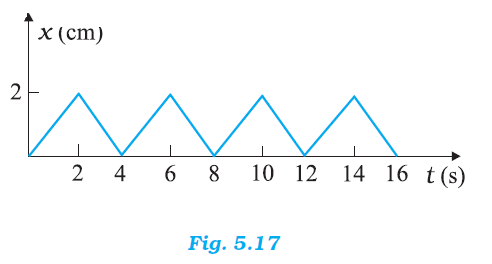## Pages

### Laws of motion NCERT Solutions Class 11 Physics - Solved Exercise Question 5.24

Question 5.24:
Figure 5.17 shows the position-time graph of a body of mass 0.04 kg. Suggest a suitable physical context for this motion. What is the time between two consecutive impulses received by the body? What is the magnitude of each impulse?Solution:
A ball rebounding between two walls located between at x = 0 and x = 2 cm; after every 2 s, the ball receives an impulse of magnitude 0.08 × 10–2 kg m/s from the walls
The given graph shows that a body changes its direction of motion after every 2 s. Physically, this situation can be visualized as a ball rebounding to and fro between two stationary walls situated between positions x = 0 and x = 2 cm. Since the slope of the x-t graph reverses after every 2 s, the ball collides with a wall after every 2 s. Therefore, ball receives an impulse after every 2 s.
Mass of the ball, m = 0.04 kg
The slope of the graph gives the velocity of the ball. Using the graph, we can calculate initial velocity (u) as:u = (2 - 0) X 10-2 / (2 - 0)  =  10-2 m/s
Velocity of the ball before collision, u = 10–2 m/s
Velocity of the ball after collision, v = –10–2 m/s
(Here, the negative sign arises as the ball reverses its direction of motion.)
Magnitude of impulse = Change in momentum
= | mv - mu |
= | 0.04 (v - u) |
= | 0.04 (-10-2 - 10-2) |
= 0.08 X 10-2 kg m/s